Nonhyperbolic reflection moveout of-waves: An overview and comparison of reasonsNext: Vertically heterogeneous VTI model Up: VERTICAL HETEROGENEITY Previous: VERTICAL HETEROGENEITY

## Vertically heterogeneous isotropic model

Nonhyperbolicity of reflection moveout in vertically heterogeneous isotropic media has been extensively studied using the Taylor series expansion in the powers of the offset (Bolshykh, 1956; Taner and Koehler, 1969; Al-Chalabi, 1973). The most important property of vertically heterogeneous media is that the ray parameterdoes not change along any given ray (Snell's law). This fact leads to the explicit parametric relationships(19)(20)

where(21)

Straightforward differentiation of parametric equations (19) and (20) yields the first four coefficients of the Taylor series expansion(22)

in the vicinity of the vertical zero-offset ray. Series (22) contains only even powers of the offset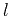because of the reciprocity principle: the pure-mode reflection traveltime is an even function of the offset. The Taylor series coefficients for the isotropic case are defined as follows:(23)(24)(25)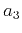(26)

where(27)(28)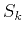(29)

Equation (24) shows that, at small offsets, the reflection moveout has a hyperbolic form with the normal-moveout velocity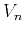equal to the root-mean-square velocity. At large offsets, however, the hyperbolic approximation is no longer accurate. Studying the Taylor series expansion (22), Malovichko (1978) introduced a three-parameter approximation for the reflection traveltime in vertically heterogeneous isotropic media. His equation has the form of a shifted hyperbola (Castle, 1988; de Bazelaire, 1988):(30)

If we set the zero-offset traveltimeequal to the vertical traveltime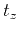, the velocityequal to, and the parameter of heterogeneityequal to, equation (30) guarantees the correct coefficients,, andin the Taylor series (22). Note that the parameteris related to the varianceof the squared velocity distribution, as follows:(31)

According to equation (31), this parameter is always greater than unity (it equals 1 in homogeneous media). In many practical cases, the value oflies betweenand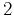. We can roughly estimate the accuracy of approximation (30) at large offsets by comparing the fourth term of its Taylor series with the fourth term of the exact traveltime expansion (22). According to this estimate, the error of Malovichko's approximation is(32)

As follows from the definition of the parameters[equations (29)] and the Cauchy-Schwartz inequality, the expression (32) is always nonnegative. This means that the shifted-hyperbola approximation tends to overestimate traveltimes at large offsets. As the offset approaches infinity, the limit of this approximation is(33)

Equation (33) indicates that the effective horizontal velocity for Malovichko's approximation (the slope of the shifted hyperbola asymptote) differs from the normal-moveout velocity. One can interpret this difference as evidence of some effective depth-variant anisotropy. However, the anisotropy implied in equation (30) differs from the true anisotropy in a homogeneous transversely isotropic medium [see equation (1)]. To reveal this difference, let us substitute the effective values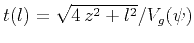,,, andinto equation (30). After eliminating the variablesand, the result takes the form(34)

If the anisotropy is induced by vertical heterogeneity,. Those inequalities follow from the definitions of,,, and the Cauchy-Schwartz inequality. They reduce to equalities only when velocity is constant. Linearizing expression (34) with respect to Thomsen's anisotropic parametersand, we can transform it to the form analogous to that of equation (5):(35)

Figure 3 illustrates the difference between the VTI model and the effective anisotropy implied by the Malovichko approximation. The differences are noticeable in both the shapes of the effective wavefronts (Figure 3a) and the moveouts (Figure 3b).nmofrz1,nmofrz2
Figure 3.
Comparison of the wavefronts (a) and moveouts (b) in the VTI (solid) and vertically inhomogeneous isotropic media (dashed). The values of the effective vertical, horizontal, and NMO velocities are the same in both media and correspond to Thomsen's parametersand.In deriving equation (35), we have assumed the correspondence(36)

We could also have chosen the value of the parameter of heterogeneitythat matches the coefficientgiven by equation (25) with the corresponding term in the Taylor series (13).Then, the value ofis (Alkhalifah, 1997)(37)

The difference between equations (36) and (37) is an additional indicator of the fundamental difference between homogeneous VTI and vertically heterogeneous isotropic media. The three-parameter anisotropic approximation (12) can match the reflection moveout in the isotropic model up to the fourth-order term in the Taylor series expansion if the value ofis chosen in accordance with equation (37). We can estimate the error of such an approximation with an equation analogous to (32):(38)

The difference between the error estimates (32) and (38) is(39)

For usual values of, which range fromto, the expression (39) is positive. This means that the anisotropic approximation (12) overestimates traveltimes in the isotropic heterogeneous model even more than does the shifted hyperbola (30) shown in Figure 3b. Below, we examine which of the two approximations is more suitable when the model includes both vertical heterogeneity and anisotropy.Nonhyperbolic reflection moveout of-waves: An overview and comparison of reasonsNext: Vertically heterogeneous VTI model Up: VERTICAL HETEROGENEITY Previous: VERTICAL HETEROGENEITY

2014-01-27